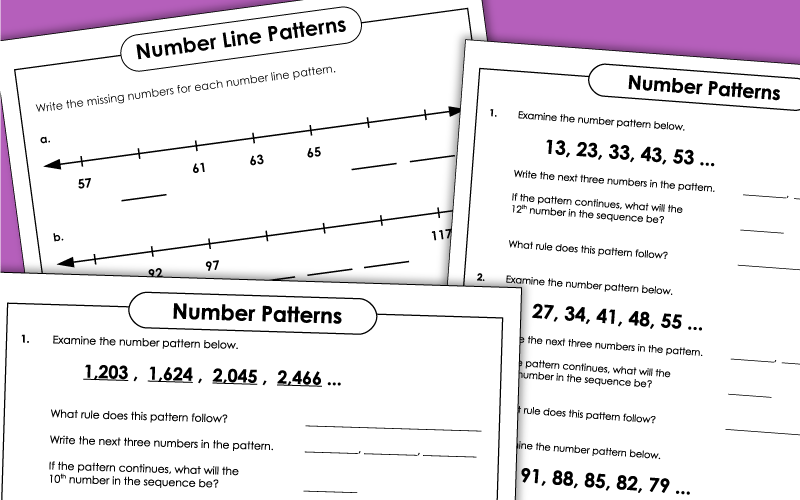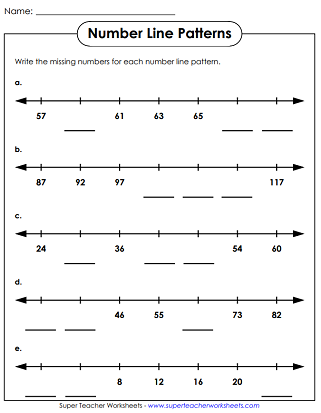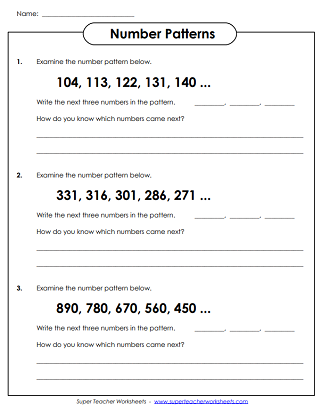# Number Patterns

For each number pattern set, students must figure out the rule for continuing the pattern.## Basic (1-2)

The number patterns require students to count by 2s, add 10, and count by 3s.

## Intermediate (2-3)

Determine which numbers come next in the pattern and explain the rule.  This worksheet has 2-digit number lists.
Describe the rule used in each number pattern and list the three numbers that come next.  The lists feature 3-digit numbers.
Continue each number pattern and answer the questions.
Write the missing numbers on each number line.

Continue the number patterns and describe the rule.  The numbers on this worksheet are in the thousands.
In and Out Boxes

These worksheets are similar to number patterns in that students must find the correct rule.  They are designed as input/output boxes.

Picture Patterns

Determine which pictures come next in each pattern shown.

Skip Counting Worksheets

Worksheets for teaching students to skip count by 2s, 3s, 4s, 5s, 10s, 25s, and 100s.

## Sample Worksheet ImagesMy Account
Site Information